# NCERT Solutions for Class 9 Maths Chapter 13 Surface Areas and Volumes Ex 13.8

NCERT Solutions for Class 9 Maths Chapter 13 Surface Areas and Volumes Ex 13.8 are part of NCERT Solutions for Class 9 Maths. Here we have given NCERT Solutions for Class 9 Maths Chapter 13 Surface Areas and Volumes Ex 13.8.

 Board CBSE Textbook NCERT Class Class 9 Subject Maths Chapter Chapter 13 Chapter Name Surface Areas and Volumes Exercise Ex 13.8 Number of Questions Solved 10 Category NCERT Solutions

## NCERT Solutions for Class 9 Maths Chapter 13 Surface Areas and Volumes Ex 13.8

Question 1.
Find the volume of a sphere whose radius is
(i) 7 cm
(ii) 0.63 cm
Solution:
(i) We have, r = 7 cm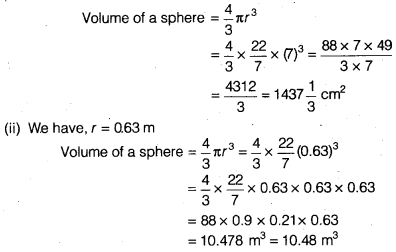Question 2.
Find the amount of water displaced by a solid spherical ball of diameter
(i) 28 cm
(ii) 0.21 m
Solution:
(i) We have, d = 28 cm
⇒ r = 14 cm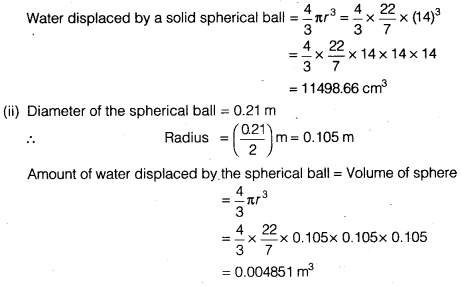Question 3.
The diameter of a metallic ball is 4.2 cm. What is the mass of the ball, if the density of the metal is 8.9 g per cm3?
Solution:
We have, d = 4.2 cm ⇒ r = 2.1 cm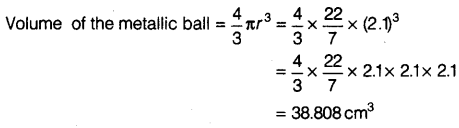The density of the metal per cm3 = 8.9 g
The density of the metal 38.808 cm3 = 38.808 x 8.9 g = 345.39 g
Hence, the mass of the ball = 345.39 g

Question 4.
The diameter of the Moon is approximately one-fourth of the diameter of the Earth. What fraction of the volume of the Earth is the volume of the Moon?
Solution:
Let diameter of the Earth is d3.
We have, diameter of Moon (d1) = $$\frac { 1 }{ 4 }$$ diameter of the Earth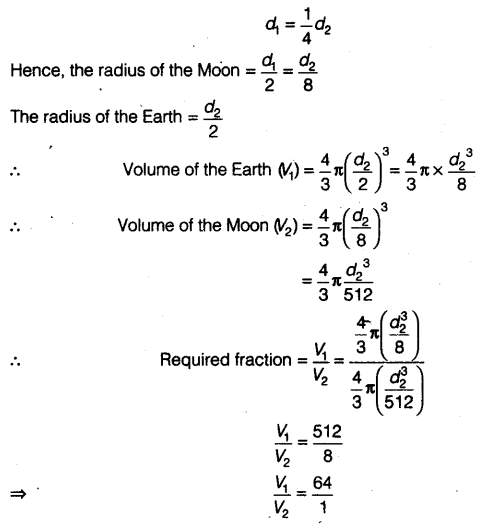Question 5.
How many litres of milk can a hemispherical bowl of diameter 10.5 cm hold?
Solution:
Diameter = 10.5 cm
⇒ Radius, r = 5.25 cm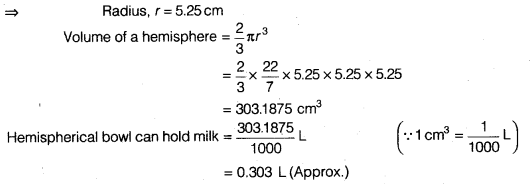Question 6.
A hemispherical tank is made up of an iron sheet 1 cm thick. If the inner radius is 1 m, then find the volume of the iron used to make the tank.
Solution:
Inner radius (r1) = 1 m -100 cm
Outer radius (r2) = (100 + 1)cm ( ∵ Inner radius + Thickness)
= 101 cm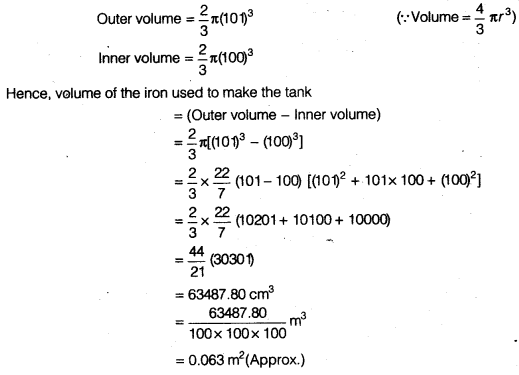Question 7.
Find the volume of a sphere whose surface area is 154 cm2.
Solution:
We have, surface area of a sphere = 154 cm2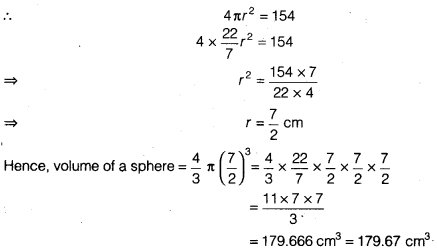Question 8.
A dome of a building is in the form of a hemisphere. From inside, it was white washed at the cost of ₹498.96. If the cost of white washing is ₹2.00 per square metre, find the
(i) inside surface area of the dome,
(ii) volume of the air inside the dome.
Solution:
Cost of white washed = ₹ 498.96
Cost of white washing per square metre = ₹ 2.00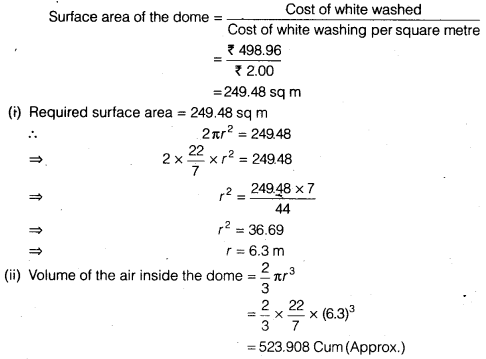Question 9.
Twenty seven solid iron spheres, each of radius r and surface area S are melted to form a sphere with surface area S’. Find the
(i) radius r’ of the new sphere,
(ii) ratio of S and S’.
Solution: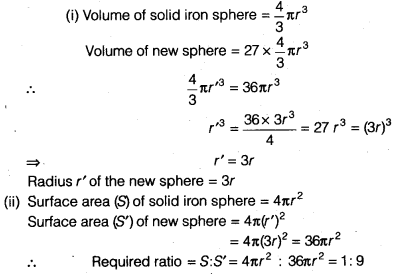Question 10.
A capsule of medicine is in the shape of a sphere of diameter 3.5 mm. How much medicine (in mm3) is needed to fill this capsule?
Solution:
We have, diameter = 3.5 mm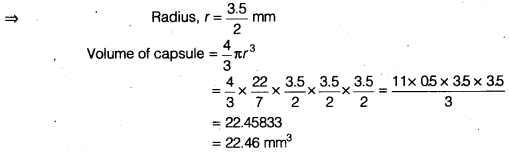We hope the NCERT Solutions for Class 9 Maths Chapter 13 Surface Areas and Volumes Ex 13.8 help you. If you have any query regarding NCERT Solutions for Class 9 Maths Chapter 13 Surface Areas and Volumes Ex 13.8, drop a comment below and we will get back to you at the earliest.